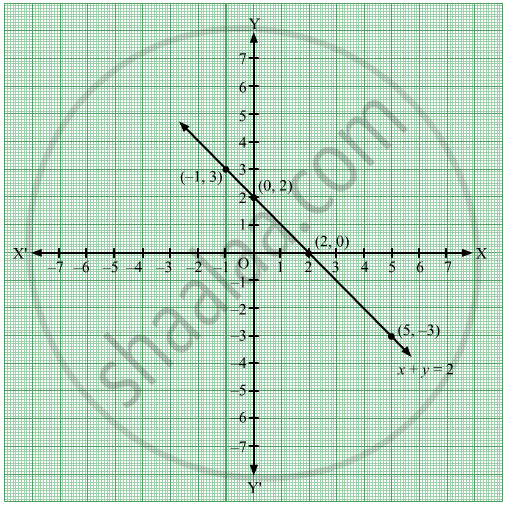Advertisement Remove all ads

# Draw the Graph of the Equation Given Below - Geometry

Graph

Draw the graph of the equation given below

x + y = 2

Advertisement Remove all ads

#### Solution

The equation of the given line is x + y = 2.
x + y = 2
⇒ y = 2 − x          .....(1)
Putting x = 0 in (1), we get
y = 2 − 0 = 2
Putting x = 2 in (1), we get
y = 2 − 2 = 0
Putting x = −1 in (1), we get
y = 2 − (−1) = 2 + 1 = 3
Putting x = 5 in (1), we get
y = 2 − 5 = −3
These values can be represented in the table in the form of ordered pairs as follows:

 x 0 2 -1 5 y 2 0 3 -3 (x , y) (0 , 2) (2 , 0) (-1 , 3) (5 , -3)

Plot these points on the graph paper.The line is the graph of the equation x + y = 2.

Concept: Graphs of Linear Equations
Is there an error in this question or solution?
Advertisement Remove all ads

#### APPEARS IN

Balbharati Mathematics 2 Geometry 9th Standard Maharashtra State Board
Chapter 7 Co-ordinate Geometry
Practice Set 7.2 | Q 9.1 | Page 98
Advertisement Remove all ads
Advertisement Remove all ads
Share
Notifications

View all notifications

Forgot password?